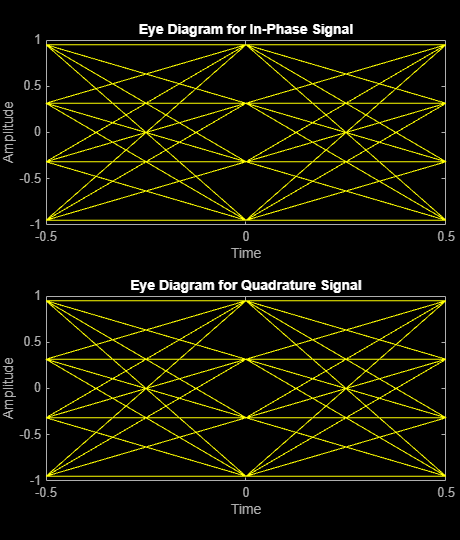# Reduce ISI Using Raised Cosine Filtering

Employ raised cosine filtering to reduce inter-symbol interference (ISI) that results from a nonlinear amplifier.

Initialize a simulation variable for modulation order.

`M = 16; % Modulation order`

Create square root raised cosine filter objects.

```txfilter = comm.RaisedCosineTransmitFilter; rxfilter = comm.RaisedCosineReceiveFilter;```

Create a memoryless nonlinearity System object™ to introduce nonlinear behavior to the modulated signal. Using name-value pairs, set the `Method` property to `Saleh model` to emulate a high power amplifier.

```hpa = comm.MemorylessNonlinearity('Method','Saleh model', ... 'InputScaling',-10,'OutputScaling',0);```

Generate random integers and apply 16-QAM modulation.

```x = randi([0 M-1],1000,1); modSig = qammod(x,M,'UnitAveragePower',true);```

Plot the eye diagram of the modulated signal. At time 0, there are three distinct "eyes" for 16-QAM modulation.

`eyediagram(modSig,2)`Amplify the modulated signal using `hpa`.

`txSigNoFilt = hpa(modSig);`

Plot the eye diagram of the amplified signal without RRC filtering. At time 0, there are multiple eyes. This is a result of inter-symbol interference from the nonlinear amplifier.

`eyediagram(txSigNoFilt,2)`Filter the modulated signal using the RRC transmit filter.

`filteredSig = txfilter(modSig);`

Release `hpa` and amplify the filtered signal. The `release` function is needed because the input signal dimensions change due to filter interpolation.

```release(hpa) txSig = hpa(filteredSig);```

Filter `txSig` using the RRC matched receive filter.

`rxSig = rxfilter(txSig);`

Plot the eye diagram of the signal after the application of the receive filter. There are once again three distinct eyes as the matched RRC filters mitigate ISI.

`eyediagram(rxSig,2)`